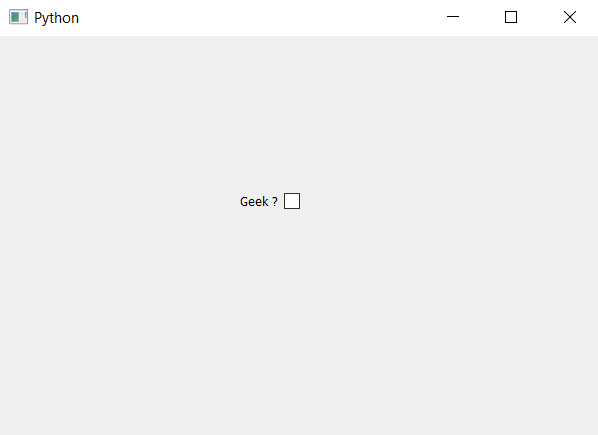# PyQt5 – Check Box with Indicator at right side

• Last Updated : 22 Apr, 2020

In this article we will see how to set the indicator to the right of check box. Check box basically have two components first is indicator and other is the label by default indicator is at the left hand side. In order to make the indicator at the right hand side we have to change its layout direction from left to right side.

In order to do this we will use `setLayoutDirection` method.

Syntax : checkbox.setLayoutDirection(Qt.RightToLeft)

Argument : It takes Qt object as argument.

Action performed : It will change the layout of check box.

Below is the implementation.

 `# importing libraries``from` `PyQt5.QtWidgets ``import` `*` `from` `PyQt5 ``import` `QtCore, QtGui``from` `PyQt5.QtGui ``import` `*` `from` `PyQt5.QtCore ``import` `*` `import` `sys`` ` ` ` `class` `Window(QMainWindow):`` ` `    ``def` `__init__(``self``):``        ``super``().__init__()`` ` `        ``# setting title``        ``self``.setWindowTitle(``"Python "``)`` ` `        ``# setting geometry``        ``self``.setGeometry(``100``, ``100``, ``600``, ``400``)`` ` `        ``# calling method``        ``self``.UiComponents()`` ` `        ``# showing all the widgets``        ``self``.show()`` ` `    ``# method for widgets``    ``def` `UiComponents(``self``):`` ` `        ``# creating the check-box``        ``checkbox ``=` `QCheckBox(``'Geek ?'``, ``self``)`` ` `        ``# setting geometry of check box``        ``checkbox.setGeometry(``200``, ``150``, ``100``, ``30``)`` ` `        ``# changing position of indicator``        ``checkbox.setLayoutDirection(Qt.RightToLeft)`` ` ` ` `# create pyqt5 app``App ``=` `QApplication(sys.argv)`` ` `# create the instance of our Window``window ``=` `Window()`` ` `# start the app``sys.exit(App.``exec``())`

Output :My Personal Notes arrow_drop_up